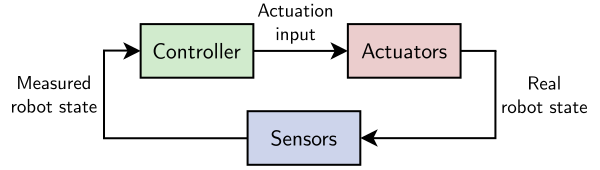# What is a controller?

There is a lot of software distributed on a robot. The piece of it that decides what to do next is called the controller.

## Feedback loop

In the general sense, robot components can be classified in three categories: actuation ("the plant", sometimes simply called "the robot"), sensing and control. A controller is, by definition,anything that turns robot outputs (a.k.a. "states", for instance joint positions and velocities) into new robot inputs (a.k.a. "controls", for instance joint accelerations or torques). Robot outputs are not known perfectly but measured by sensors. Putting these three components together yields the feedback loop:If we take the simple example of a velocity-controlled point mass, the input of your robot is a velocity and its output is a new position. A controller is then any piece of software that takes positions as inputs and outputs velocities. Under the hood, the controller may carry out various operations such as trajectory planning or PID feedback. For instance, a model predictive controller computes a desired future trajectory of the robot starting from its current (measured) state, then extracts the first controls from it and sends it to the robot.

## Example of HRP-4

The HRP-4 humanoid robot from Kawada Industries is a position-controlled robot.

• Inputs: desired joint positions
• Outputs: joint angle positions + position and orientation of the robot with respect to the inertial frame (a.k.a. "free-flyer" transform)

Being a mobile robot, it is underactuated, meaning its state has a higher dimension than its inputs. Measurements of the robot state are carried out by rotary encoders for joint angles and using an IMU for the free-flyer transform. A controller for HRP-4 therefore takes as inputs the robot's state (joint positions + position and orientation with respect to the inertial frame) and outputs a new set of desired positions.

## Q & A

How to achieve velocity/acceleration control on a position-controlled robot?

Sending successive joint angles along a trajectory with the desired velocities or accelerations, and relying and the robot's stiff position tracking.

How to control the underactuated position of the robot in the inertial frame?

Using contacts with the environment and admittance control.

## To go further

There are many references to pick from in the field of control theory. For an introduction, you can check out Quang-Cuong Pham's lecture notes on control theory.

Pages of this website are under the CC-BY 4.0 license.